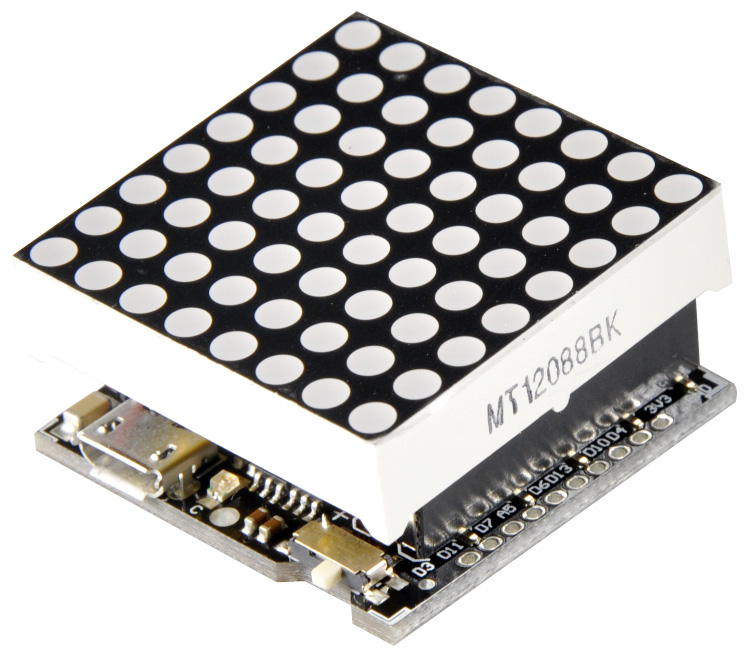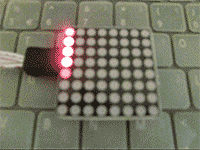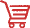# 8*8_Matrix_SKU_DFR0240-DFRobot## Instruction

A brand new way to play with 8*8 matrix! Put your board aside after uploading. This small matrix has a quicker & easier way of displaying what you want. Tiny, portable, with microcontroller, display & power supply included, making it more convenienient to play with. You can programme whatever you want to display according to the sample codes in Wiki page.For example, a beating heart, a smile face, or even a slot machine. It can be a gift to your girlfriend, children, the choice is up to you. No need to connect other jumper cables & microcontroller. Find this cool little blittering dice in the bar, in a party... Now it's your turn to give it a new life.

## Specification

• MCU: Atmega8
• Led color: red
• Programmable hang decorations
• Charging circuit build in
• Lipo battery x1
• Xbee socket x1
• 8*8 matrix module x1
• Size: 32x32mm

### Sample Code

Library for sample code

• Put the <led_8x8.h>in the same file of the sample codes
``````/**************** start of led_8x8.h ********************/
/*
*  created: 2013-06-14
*
*/
#include <Arduino.h>

const uint8_t row = {9, 8,  4, 17, 3, 10, 11,  6};
const uint8_t col = {2, 7, 19, 5, 13, 18, 12, 16};

//init 8x8 led
void led_init (void) {
for (int pin = 0; pin<8; pin++)  {
pinMode (row[pin], OUTPUT);
pinMode (col[pin], OUTPUT);
digitalWrite (row[pin], LOW);
digitalWrite (col[pin], HIGH);
}
}

//display one led
void led_xy_on (int x, int y) {
digitalWrite (row[x], HIGH);
digitalWrite (col[y], LOW);
digitalWrite (col[y], HIGH);
digitalWrite (row[x], LOW);
}

//display one led with a time
void led_xy_time (int x, int y, int time) {
for (int t=0; t<=time; t++) {
digitalWrite (row[x], HIGH);
digitalWrite (col[y], LOW);
digitalWrite (col[y], HIGH);
digitalWrite (row[x], LOW);
}
}

//display one led with a state
void led_xy_state (int x, int y, int state) {
digitalWrite (row[x], HIGH);
digitalWrite (col[y], !state);
digitalWrite (col[y], HIGH);
digitalWrite (row[x], LOW);
}

//display one picture
void led_pic (int pix) {
for (int x=0; x<8; x++)
for (int y=0; y<8; y++)
led_xy_state (x, y, pix[x][y]);
}

//loop displays a set of pictures in pix[];
void led_pix (int pix[], int pmax, int time) {
for (int p=0; p<pmax; p++)  //picture from 0 to pmax
for (int t=0; t<time; t++)  //delay
for (int x=0; x<8; x++)
for (int y=0; y<8; y++)
led_xy_state (x, y, pix[p][x][y]);
}

//open all led
void led_all_on () {
for (int i=0; i<8; i++) {
digitalWrite (row[i], HIGH);
digitalWrite (col[i], LOW);
}
}

/***************** end of led_8x8.h ***********************/``````

Sample code

``````
/*************** start of sample3.c ******************/
/*
*  created: 2013-06-14
*      loop displays a volution
*/

#include <Arduino.h>
#include "led_8x8.h"

void setup () {
led_init ();
}

void loop () {
int left = 0;
int right = 7;
int time = 500;

for (int x=0,y=0 ;left<right; left++, right--) {
for (x=left; x<=right; x++)
led_xy_time (x, y, time);
x--;
for (y=left; y<=right; y++)
led_xy_time (x, y, time);
y--;
for (x=right; x>=left; x--)
led_xy_time (x, y, time);
x++;
for (y=right; y>left; y--)
led_xy_time (x, y, time);
y++;
}
}
/*************** end of sample3.c ******************/``````

## More DocumentsGet 8*8 Matrix from DFRobot Store or DFRobot Distributor.

Turn to the Top